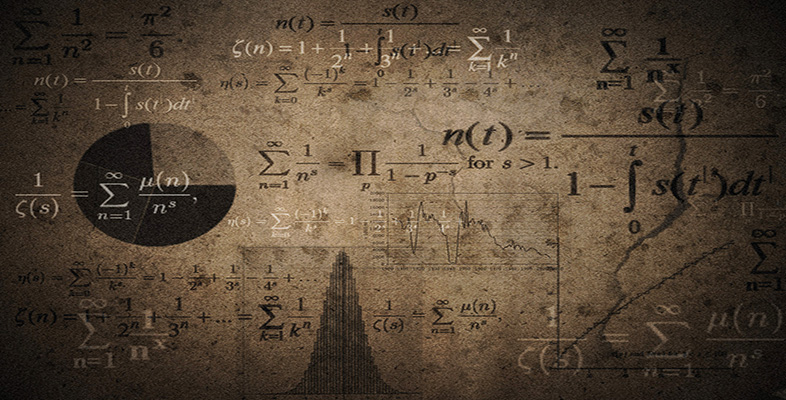Language, notation and formulas

This free course is available to start right now. Review the full course description and key learning outcomes and create an account and enrol if you want a free statement of participation.

Free course

# 2.8.1 Try some yourself

## Activity 9

What do the following mean?

• (a) (5 + 8)/(4 − 2)

• (b) 5 + 8/4 − 2

• (c) (4 + 5)(5 − 2)

• (d)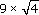• (e)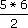• (f)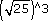• (g) (12 − 9)−1

• (a) Add 5 and 8, and then divide the result by the difference between 4 and 2. (This gives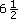.)

• (b) Add 5 to the division 8/4, and then subtract 2. (This gives 5.)

• (c) Multiply the sum of 4 and 5 by the difference between 5 and 2. (This gives 27.)

• (d) Multiply 9 by the square root of 4. (This gives 18.)

• (e) Multiply 5 by 6, and then divide by 2. (This gives 15.)

• (f) The cube of the square root of 25. (That is, 125.)

• (g) The reciprocal of 12 minus 9. (That is,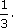)

## Activity 10

What do these symbols mean?

• (a) =

• (b) <

• (c) >

• (d)• (a) = means ‘equals’ or ‘equal to’ or ‘which equals’.

• (b) < means ‘less than’ or ‘which is less than’.

• (c) > means ‘greater than’ or ‘which is greater than’.

• (d)means ‘is approximately equal to’.

## Activity 11

What do the following mean?

• (a) mass ≥ 10 kg

• (b) time < 2.4 × 106 h

• (c) 2/3 ≠ 0.67

• (a) The mass is greater than or equal to 10 kg.

• (b) The time is less than 2.4 million hours.

• (c) Two-thirds (or 2 divided by 3) is not equal to 0.67.

MU120_4M6